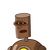# if a diagonals of parallelogram are equal then show it is a rectangle​

if a diagonals of parallelogram are equal then show it is a rectangle​

### 1 thought on “if a diagonals of parallelogram are equal then show it is a rectangle​”

1.□ ABCD is a parallelogram

consider Δ ACD and Δ ABD

AC = BD …. (given)

AB = DC …. (opposite sides of parallelogram)

∴Δ ACD ≅Δ ABD (sss test of congruence)

∠ BAD = ∠ CDA …. (cpct)